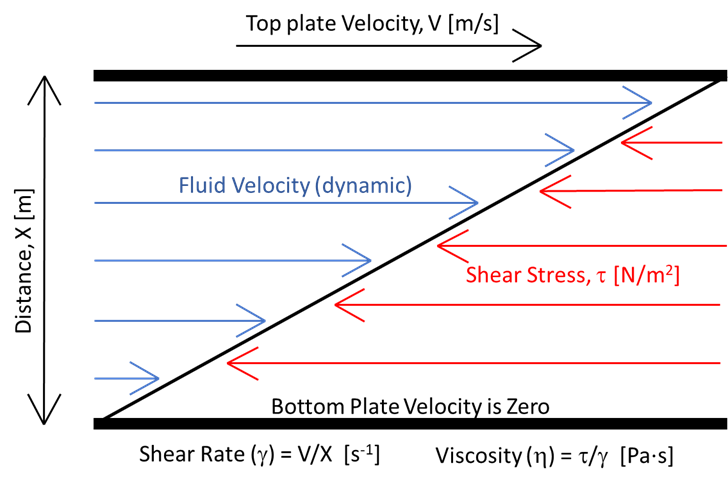Search
•AMAD, INC.

# Shear Rate and Viscosity Testing FundamentalsAnton Paar MCR 301 Rheometer

The shear rate of a fluid is defined as the rate at which layers of a fluid can move past each other. The resistance to the movement of the surfaces is a function of the viscosity of the fluid, and is known as the shear stress. Usually, the shear rate is determined using a parallel-plate rheometer, where the fluid is placed in-between two parallel discs of the same diameter. The top disc is rotated while the bottom plate remains stationary.

To visualize the “shearing effect” of the fluid, it is helpful to envision two parallel plates moving past each other. The top plate, which is in motion, has a velocity of V (m/s), the bottom plate, which is stationary, has a velocity of zero, and the distance between the plates is X (m). The shear rate is defined as the velocity of the top plate divided by the distance between plates. The shear stress is caused by the difference in velocity between the plates, and the viscosity is found by dividing the shear stress by the shear rate. A visual depiction of this scenario is given below, along with the equations for shear rate and viscosity.Figure 1: Visualization of a fluid under shear from two parallel plates, showing velocity and force directions.

Rheometers are used to measure the shear rate, shear stress, and viscosity to generate flow curve profiles. Advanced Materials and Devices, Inc. uses an Anton Paar MCR 301 Rheometer equipped with temperature control to perform rheological testing on a variety of materials ranging from low viscosity fluids to stiff elastomers. For more information on our rheological testing services click here, or check out our full line of testing services.